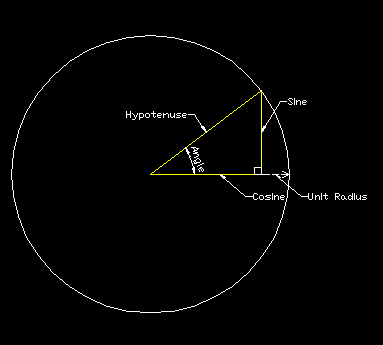### Seeing a Rotation Matrix

Written by MickD www.dcservices.net.au
One of the hardest things I found when learning about geometry and computer graphics was how to 'see' a rotation matrix and how they are used, they are a handy thing to have and understand in your programming toolkit for AutoCad also. I won't delve to deep into matrices or calculations, what I hope to achieve in this article is to expose a simple way for you to 'see' a rotation matrix, when I read an article on basic trig it all fell into place so that's where we'll start.

## Our use of Trigonometry

Without going into the nitty gritty of it, trig is basically about triangles, 'right' triangles to be exact and the thing they didn't teach us at school (our school anyway) was that the basic elements of trig, being sine, cosine and tangent, were all based around angles within a unit circle! This is a very important concept to remember and will be used later, so pay attention ;) (A unit circle is simply a circle with a radius of 1, a single unit.
Looking at Figure 01 above we can see that sine is on the opposite side of our angle at the centre of the circle, and cosine is adjacent to our angle. All basic stuff we remember from school but keep the circle in your head when thinking about it, this is what makes 'seeing' a rotation matrix easier as you will soon see.

### Where does sine and cosine come from?

This was a question I had since primary school, all I new back then is that you had a table full of numbers and angles (or in your calculator these days) to refer to that some genius must have made up in his spare time to help us get through math class (btw, thanks ;) ). But basically the numbers in the table are made from the sine of each angle in a unit circle. Now, when you place your triangle in a circle, as in our figure above, you can see in Figure 02 that if you double the angle to make another triangle, the 2 triangle's sine's make a 'chord' of the circle, with this we can see that the sine of an angle is exactly half of the chord of double the angle of a unit circle. Easy 'ey!Figure 01. Showing the elements involved in trigonometry calculations.Figure 02. Showing the chord of a circle used to calculate sine

## The Rotation Matrix

Our base coordinate system is made from the 3 unit vectors x, y and z (in 3space anyways) and when we use a rotation matrix we actually rotate these 3 vectors then calculate our points to draw our objects based on this new coordinate system. Sorry to just breeze over vectors but I'll be here all day if I try to explain,( maybe another time ;)) but basically a unit vector has a value of 1 or 1 unit of measurement and to rotate them we need our matrix.
Going back to our trig discussion, have a look at the hypotenuse and our radius, they are both unit vectors right? and if you look a bit harder you can see that if we rotated our radius vector by our angle, it gives us the hypotenuse!
Now, have a look at the figure 03 and you can see that I have drawn a unit circle on the xy plane of our coordinate system as that is the plane we need to rotate to move our x and y around the z axis. I have also drawn the new coordinate system that has been rotated by an angle and if we draw the sine and cosine in we have formed our triangle and we can now 'see' where we need to use sine and cosine in our matrix calculations.
Here is the matrix for an anti-clockwise rotation around the 'z' axis, try to do the x and y rotation axes yourself, it should only take a few minutes now you can 'see' how they work!
```* = angle
| cos*   -sin*    0 |
| sin*    cos*    0 |
| 0        0      1 |```Figure 03. Showing the rotation of the coordinate system around the 'z' axis.

Need drafting work?
We are having great success with Freelancer.com. With this website we can win all types of drafting work. People from all over the world are looking for freelancers skilled in AutoCAD, Revit, Civil 3D and Microstation, are you one of those people? If so you can live the freelancer lifestyle! and work from any location you want too. Freelancer.com, it works really well - we like it - and we believe you will too.